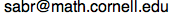## Math Explorer's Club Tips for Mental Computations

Introduction
Lesson 2: Subtraction
Lesson 3: Multiplication
Lesson 4: Division
Lesson 5: Calendar computations
Lesson 6: Guessing a number and other tricks

We are going to study some ways to do computations fast! We will look at several tricks that will surely impress your friends.

In the activities you can try to see why the tricks work. For this we need to recall the decimal expansion of a number. For instance, the decimal expansion of 246 is 2 × 100 + 4 × 10 + 6.

Here we use the notation ab to indicate a the number we get by concatenating two integers a and b. So ab is NOT the multiplication of a and b; that will be denoted by a × b. So if a = 12 and b = 45, then ab = 1245. More often than not, a and b will be digits.

We hope you enjoy the tips presented here. Comments are welcomed, and can be directed to# References

There are many books that contain information on tricks to do mental computations. Here are the ones that were used to create these lessons.
1. Secrets of Mental Math by Arthur Benjamin and Michael Shermer.
2. Short-cut Math by Gerard W. Kelly.
3. Speed Mathematics by Bill Handley.
4. Mathematical Recreations, second revised edition, by Maurice Kraitchik.
Cornell University, Department of Mathematics